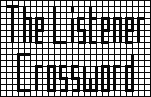## Listener Crossword 4036: Solution NotesBack to 2009 menu

## Base Jog by Brimstone

### Detailed Solution

Many clues “read” as words or phrases relevant to baseball, the theme of the puzzle. Clue C Down reads as TUNGSTEN, and is a tribute to the setter of an earlier numerical puzzle related to baseball: No 3290, BR’s Home Base by Wolfram. Wolfram is another name for Tungsten.

As for most such puzzles, there are other methods of approach but the following is broadly the path intended by the setter.

1. There are only four numbers which have across and down clues. By considering the lengths of answers it can be fairly quickly established that A=7, B=2, H=4 and U=9.
2. Because 4 across, BUR+Y, is only three digits and BU = 29 = 512 it follows that R=1 and Y=0 and 4 across = 512. Also Z=25 (now the only letter without a clue).
3. 9 down, HUH is now known, as 144. HU–N–B+UB = 50 and it follows that M=19, since it cannot be entered at 7 down.
4. 2 down = AS = ?1? with A=7 and the length of the across entry for S gives S=17, 2 down = 119, 2 across = 106, 7 down = 43.
5. Checking the lengths of products of unused numbers shows that when three are involved the answer is too long for a 2-digit entry. Hence, from its answer length, O=5 or 22 but part of clue for P, ie, O, implies that O=5 (22 would be too big). P across starts with 32.
6. Similarly the UE = 9E term implies E=3, as 2, 4 and 5 are already used.
7. From down clues for T and H (=4) it follows T=8 and thus 8 down = 333, 5 across = 53 and 4 down begins 5632.
8. There are several different paths from here. For example, consideration of 7 across gives D=15. Consideration of 9 across and J across, using available across value, gives J=11 and thus 11 across = 231. 9 across also gives G=13 implying 3 down is 657554 and 9 across = 133705.
9. From 1 down, C=10. The end of 7 across gives L=18.
10. 17 across ends 255. P is long and so we must have P=20. K is the only short entry, so K=22 and the entry is 83. 13 across ends 22.
11. Down sizes give N=6 and its 21 in the middle shows W=21. Most of 20 across is filled; its ending gives X=14.
12. 14 down shows 91 + 2I = ??3, with I = 6, 11, 16, etc. The only possibility is 123, with I=16. The only down left is F=12. 6 down ends 216, showing Q=23 (476) and V=24 (477).
13. All letter values are now known and the preliminary grid can be completed.

Now, 18 digits can be changed to spell Babe Ruth and Henry Aaron, in the outline of a home run at baseball. On April 8, 1974, Aaron hit his 715th major league home run, eclipsing Ruth’s long-held record of 714. The title of the puzzle is BASE (home) = 2x7x17x3 = 714 and JOG (run) = 11x5x13 = 715. Entering 1 in the central cell completed these two numbers diagonally in the grid. Mathematicians noted these were very unusual numbers with several interesting properties including the fact that 714 = 2x3x7x17, 715 = 5x11x13 and 2+3+7+17 = 5+11+13 = 29; numbers with these properties have since become known as Ruth-Aaron pairs. Aaron’s record was eclipsed on August 7, 2007 by Barry Bonds.

Back to 2009 menu